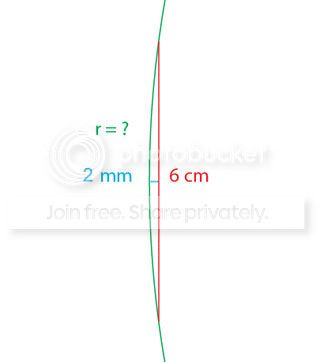I have a lens (magnifying glass) and I want to calculate the radius of the curvatures on its sides. The lens in question

diameter of the lens = 6 cm
thickness at center = 7 mm
thickness at edge = 3mmIf we were to simplify itHow can I calculate the radius? You don't have to work with these values if you want to show an example calculation, but I'd appreciate if you did.

• Isn't it $2$mm instead of $1.5$mm? Because, you know, $\frac{7-3}{2}=2$? Aug 21, 2014 at 21:49
• Yea, sorry. Just noticed. Updated it. Aug 21, 2014 at 21:51

Refer to this diagram:We have $(r-h)^2+x^2=r^2$. Expanding this we get $$r^2-2hr+h^2+x^2=r^2$$ and after subtracting $r^2$ from both sides and rearranging $$r=\frac{h^2+x^2}{2h}$$ Note that $x$ is only half the length of the chord.

With your specific figures being $h=2mm$ and $x=30mm$ you should get $r=226mm$ corresponding to $r=22.6cm$. The following diagram supports this:HINT

The $6$cm segment is a chord of the circle. Also, the $2$mm line is perpendicular, and the arc is symmetric respect to that segment, so it's obvious that it lies on the diameter.

Then, as we know, any two chords $\overline{AB}$ and $\overline{CD}$ that intersect on a pont $X$, will hold the equality

$$|\overline{AX}|\cdot |\overline{XB}|=|\overline{CX}|\cdot |\overline{XD}|$$

HINT 2

One of the chords can be the diameter!

HINT 3

In your case, $|\overline{AX}|=|\overline{XB}|=30$mm, and as the other chord is the diameter,

$$|\overline{CX}|=2\mathrm{mm}$$ $$|\overline{XD}|=2r-2\mathrm{mm}$$